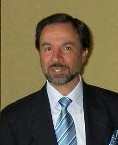Enter the content which will be displayed in sticky bar

# MemberDiego Jos? Arturo Sa
local time: 2022-05-17 06:37 (-05:00 )
Diego Jos? Arturo Sa (Abstracts)
Titles
• [Updated 5 years ago]
• [Updated 9 years ago]
• [Updated 5 years ago]
• Mass, Energy, Momentum (2008) [Updated 1 decade ago]
• Fourvector Algebra (2007) [Updated 5 years ago]
• [Updated 4 years ago]
Abstracts Details
• [Updated 5 years ago]

Physicists have the imperative need to figure out what they are talking about. Reality is not anymore the familiar and recognizable matter it used to be in previous centuries. Moreover, the newly discovered phenomena and its behavior, such as electrons, atoms, speed of light, Maxwell's equations and others, have forced the investigators to develop new theories that, basically in the twentieth century, attempted to provide more accurate models of reality than the ones available at that moment, such as Galilean Relativity and Newtonian Mechanics. Regrettably, those new theories, such as Special Relativity and Quantum Theory, together with the Standard Model, have not provided an integral vision of the world and have become somehow paradoxical, disconnected and incompatible with each other. The purpose of this paper is to suggest a model of reality based on electromagnetism, which has a high probability of being correct and carries the promise of integrating all branches of Physics. In addition, it proposes a completely new mindset of physicist for thinking and believing about the different concepts that have appeared and continue appearing in physics.

• [Updated 9 years ago]

The theoretical and experimental reasons leading to the unification of electromagnetism and gravitation are discussed. The conservation of momenta is explored and is shown that it applies in the same way both to the binding of the electron to the nucleus of an atom as well as to the planets around the sun. This idea, in fact, unifies gravitation with electromagnetism. A model based on our theory is proposed, which solves the Pioneer anomaly problem.

• [Updated 5 years ago]

A new mathematical structure intended to formalize the classical 3D and 4D vectors is briefly described. This structure is evidenced to be more appropriate, for its use in Physics and the sciences in general, than any of the other mathematical structures of geometric origin, such as the Hamilton (or Pauli or Dirac) quaternions, tensors, geometric algebra (GA) and space-time algebra (STA). The application of four-vectors in electromagnetism is demonstrated, where current concepts are reproduced, in some cases, corrected, in other cases, and new concepts are discovered, such as the following: It is suggested the need of an electromagnetic scalar, the Lienard and Wiechert potentials are suggested to be incorrect and also to have an incorrect origin, new equations for the handling of energy-momentum are proposed with which it is proved that mass and momentum have to satisfy the wave equation. Several other physical variables are also proved to satisfy the wave equation, which gives a strong argument to conclude that our universe is of electromagnetic constitution. Maxwell's equations are reduced to a simple four-vector equation. As a byproduct, new values and units for the dielectric permittivity and magnetic permeability of vacuum are proposed. Then the electric and magnetic units are expressed only in terms of mechanical units so there is no need for the former.

• Mass, Energy, Momentum (2008) [Updated 1 decade ago]

The author offers the interpretation that the mass is a relativistic invariant. Next it is evidenced that the formula for the momentum four-vector cannot beapplied, given the current interpretations of Physics, neither to particles nor to photons, and that the photons should have mass. Then it is proposed a postulate about the electromagnetic constitution of matter, which gets rid of these problems. Finally, the validity and applicability of the equivalence of mass and energy is evaluated and is confirmed the requirement that the constitution of mass must be electromagnetic.

• Fourvector Algebra (2007) [Updated 5 years ago]

The algebra of fourvectors is described. The fourvectors are more appropriate than the Hamilton quaternions for its use in Physics and the sciences in general. The fourvectors embrace the 3D vectors in a natural form. It is shown the excellent ability to perform rotations with the use of fourvectors, as well as their use in relativity for producing Lorentz boosts, which are understood as simple rotations.

The probability distribution function for thermodynamics and econophysics is obtained by solving an equilibrium equation. This approach is different from the common one of optimizing the entropy of the system or obtaining the state of maximum probability, which usually obtains as a result the Boltzmann distribution. The Gamma distribution is proposed as a better equation to describe the blackbody radiation in substitution of Planck's radiation equation. Also, a new form of entropy is proposed, that maintains the correct relation with the Clausius' formula.

• [Updated 4 years ago]

Some reasons are given to suggest that the interpretation of the Lorentz' transformations as if they referred to coordinates instead of to intervals could be incorrect. Besides, the usual form of such transformations, by using variables that represent finite values instead of differentials, could be another error. Later it is shown that the Lorentz contraction factor must not have the form currently accepted for it if the Lorentz contraction factor is assumed to be equal to the quotient between time differentials.

This is a slightly different version from the one presented at the NPA meeting at U. of Connecticut, Storrs, "On Lorentz Transformation". Contents changed (Arxiv moderators recommended merging with other paper).

The inequality is computed through the so-called Gini index. The population is assumed to have the variable of interest distributed according to the Gamma probability distribution. The results show that the Gini index is reduced when the population is grouped. The number of individuals in the groups is the relevant parameter, but this number does not need to be very large in order to obtain a very substantial reduction of inequality.